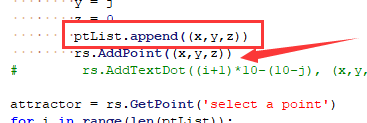# Rhpython中如何隐藏一系列点

Point3d 本身是不会显示在场景中的，目前还看不到你是用什么方法把点生成到场景中的，但只要显示在场景中就一定有 GUID。

import rhinoscriptsyntax as rs

ptList = []
for i in range(10):
for j in range(10):
x = i
y = j
z = 0
ptList.append((x,y,z))

attractor = rs.GetPoint(‘select a point’)
for i in range(len(ptList)):
distance = rs.Distance(attractor, ptList[i])

ptList2 = []
for i in range(10):
for j in range(10):
x = i + 10
y = j
z = 0
ptList2.append((x,y,z))

crvID = rs.GetObject(‘select a closed curve’, rs.filter.curve)
center = rs.CurveAreaCentroid(crvID)
for i in range(len(ptList2)):
translation = (ptList2[i] - center, ptList2[i] - center, ptList2[i] - center,)
newcurve = rs.CopyObject(crvID, translation)
rs.RotateObject(newcurve, ptList2[i], i/2)
rs.HideObject(ptList)ok好的，谢谢！